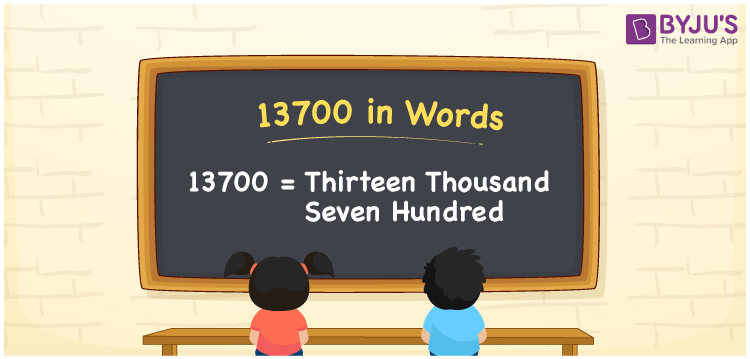# 13700 in words

13700 in words is written as Thirteen Thousand Seven Hundred. In 13700, 1 has a place value of ten thousand, 3 has a place value of thousand and 7 has a place value of hundred. The article on Place Value gives more information. The number 13700 is used in expressions that relate to money, distance, length, count and many more. For example, “Thirteen Thousand Seven Hundred Rupees was spent on buying a wooden sofa.” Another example. “I finished typing an article that had Thirteen Thousand Seven Hundred words in it”.

 13700 in words Thirteen Thousand Seven Hundred Thirteen Thousand Seven Hundred in Numerical Form 13700

## 13700 in English Words## How to Write 13700 in Words?

We can convert 13700 to words using a place value chart. This can be done as follows. The number 13700 has 5 digits, so let’s make a chart that shows the place value up to 5 digits.

 Ten thousands Thousands Hundreds Tens Ones 1 3 7 0 0

Thus, we can write the expanded form as:

1 × Ten thousand + 3 × Thousand + 7 × Hundred + 0 × Ten + 0 × One

= 1 × 10000 + 3 × 1000 + 7 × 100 + 0 × 10 + 0 × 1

= 13700

= Thirteen Thousand Seven Hundred.

13700 is the natural number that is succeeded by 13699 and preceded by 13701.

13700 in words – Thirteen Thousand Seven Hundred.

Is 13700 an odd number? – No.

Is 13700 an even number? – Yes.

Is 13700 a perfect square number? – No.

Is 13700 a perfect cube number? – No.

Is 13700 a prime number? – No.

Is 13700 a composite number? – Yes.

## Solved Example

1. Write the number 13700 in expanded form

Solution: 1 x 10000 + 3 x 1000 + 7 x 100 + 0 x 10 + 0 x 1.

Or Just 1 x 10000 + 3 x 1000 + 7 x 100.

We can write 13700 =10000 + 3000 + 700 + 0 + 0

= 1 x 10000 + 3 x 1000 + 7 x 100 + 0 x1 0 + 0 x 1.

## Frequently Asked Questions on 13700 in words

Q1

### How to write 13700 in words?

13700 in words is written as Thirteen Thousand Seven Hundred.
Q2

### State whether True or False. 13700 is divisible by 3?

False. 13700 is not divisible by 3.
Q3

### Is 13700 divisible by 10?

Yes. 13700 is divisible by 10.
Test your Knowledge on 13700 in Words